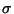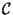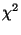Next:  My native Patterson function Up:  Pathology of GraphEnt calculations, Previous:  The GraphEnt map changes   Contents

## All my anomalous Pattersons are consistent with a uniform map''

Why : Assume for a minute that all your reflections have the same F/(F) =. Then, because for the initial (uniform) map all Fourier coefficients are identically zero (with the exception of F000), the statisticis given by===2 = N2

where N is the number of observed reflections. Now, the target value forduring the calculation is= N. If< 1.0, ie< 1.0, the uniform map will satisfy theconstraint and GraphEnt will stop immediately. Just because GraphEnt stops, does not necessarily mean that there is no signal in the data : for Gaussian noise,N is the expected value of the distributionN. This means that depending on the data, the target of the calculation could as well be significantly lower than the value aimed for by GraphEnt. I think it is worth emphasising this with an example. The following figure compares the conventional (left) and GraphEnt (right) map at the section v = 1/2 of the 20-3Å anomalous Patterson function for horse heart myoglobin crystals (dashed contour at the mean, and then every 0.5 rmsd of the whole map). The data were collected with CuKradiation and the anomalous signal comes from the iron atom of heme (this is one of the examples distributed with GraphEnt, file Myoglobin_anom_Patt_no_outliers.in).A normal run of GraphEnt with the whole data set would immediately stop with the uniformity'' message. Even after rejecting all reflections with F/(F) < 0.5, GraphEnt would still refuse to co-operate (for 615 reflections with F/(F) > 0.5, the initial--for the uniform map-- was 502.8). The map shown above could only be produced after explicitly setting the TARGet-value to 100.0 (by editing the MAXENT_AUTO.IN file). As you see, it probably worth the effort20.

Getting around it : Start GraphEnt the usual way. When the program stops with the uniformity'' message, edit the MAXENT_AUTO.IN and add a line with a new TARGet value (which should be less than the startingvalue reported by the program if the VERBose flag is set on, see page). Depending on the circumstances, you could also add a line with LIMIt 0.5 to exclude reflections with F/(F) < 0.5 (this should reduce the amount of computation required for convergence).

#### Footnotes

... effort20
I should add, however, that I am not convinced that changing the TARGetis the correct way around. To continue with the example, even if we take the expected value ofto beN - 3= 509 (ie 3away from the mean), the uniform map is still consistent with the data. The fact that there seems to be some signal in the data when we reduce the TARGet, probably points the way to over-estimated standard deviations.Next:  My native Patterson function Up:  Pathology of GraphEnt calculations, Previous:  The GraphEnt map changes   Contents
NMG, Nov 2002